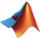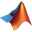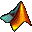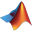84,488,480 programs installed

# The MathWorks, Inc.

### Publisher description (from The MathWorks, Inc.)

MathWorks is the leading developer of mathematical computing software for engineers and scientists
Publisher URL: www.mathworks.com

### Programs by The MathWorks

8 known major program versions, ordered by most used.

####MATLAB R2012b

 The MathWorks, Inc. 2% remove

####MATLAB R2012a Student Version 32-bit

 The MathWorks, Inc. 2% remove
Use the tools of technical inspiration -- in the classroom and throughout the industry. With MATLAB and Simulink skills, you have a brilliant future ahead. MATLAB® is a high-level language and... More about MATLAB R2012a Student Version 32-bit

####MATLAB Compiler Runtime 7.14

 The MathWorks, Inc. 6% remove

####MATLAB R2006b

 The MathWorks, Inc. 9% remove
MATLAB® is a high-level language and interactive environment for numerical computation, visualization, and programming. Using MATLAB, you can analyze data, develop algorithms, and create models and... More about MATLAB R2006b

####MATLAB R2011b 32-bit

 The MathWorks, Inc. 7% remove

#### The MathWorks Root Certificates

 The MathWorks, Inc. 1% remove

#### 2012R1_Apr MATLAB Courses

 The MathWorks, Inc. 7% remove

####MATLAB Production Server R2014b

 The MathWorks, Inc. 9% remove